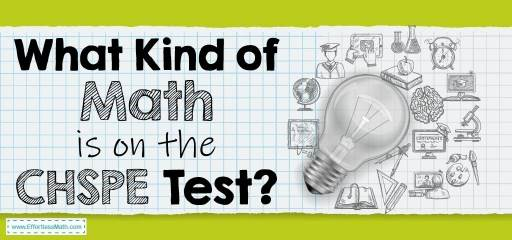# What Kind of Math Is on the CHSPE Test?The CHSPE is a standardized test for assessing the skill level of 10th-grade students in California. The purpose of this test is to help students who plan to leave high school early or go to college. Test takers who successfully pass the CHSPE test receive a Certificate of Proficiency from the California State Board of Education, equivalent to a high school diploma.

The CHSPE test has two parts: English Language Arts, Mathematics.

The math section of this test consists of 50 multiple-choice questions and assesses the knowledge of test-takers in the following areas:

• Number Sense and Operations
• Patterns, Relationships, And Algebra
• Data, Statistics, And Probability
• Geometry and Measurement

The total amount of time you have for the test is three and a half hours. Neither of the two sections of the test is timed, and you can divide the time as you like. A basic calculator is also allowed in the CHSPE Math section

In the following section, we briefly review the mathematical topics in the test:

## Number Sense and Operations

The first part of the HSPT Math test focuses on topics such as scientific notation, estimation, the order of operation, exponents, operations with real numbers and radicals, and absolute value. Your ability to figure out the numbers and possible operations between them is measured in this section.

## Patterns, Relationships, And Algebra

In this section, you should be able to show that in addition to performing algebra operations, you can also identify numerical patterns and relationships between numbers. Questions include topics such as the slope of a line, linear functions, inequalities, solving quadratic, and geometric transformations (dilation, reflection, and transformation).

## Data, Statistics, And Probability

This section is for testing your ability with statistics, data interpretation, and data analysis. In this section, you must Make inferences from data, Solve problems with permutations and combinations. Also in this section, you will be faced with some questions of Simple and compound probability.

## Geometry and Measurement

The remaining questions are about geometry and measurement, which include solid geometry and coordinates geometry. HSPT test usually includes basic geometry questions. In the geometry section, you should be familiar with Scale drawings and measurements of Perimeter, circumference, area, and volume. You also should know how to use Pythagoras’ theorem.

## FAQs:

### Is the CHSPE Math test hard?

If you take enough time to prepare for the CHSPE test, it will not be difficult for you.

### How do I study for the CHSPE Math?

How you prepare for the CHSPE Math test is very important. You can use good prep books to prepare well for the CHSPE Math test. You can also use online practice tests for further practice after studying the CHSPE exam courses.

### How is the CHSPE Test Scored?

Looking for the best resource to help you succeed on the CHSPE Math test?

## The Best Books to Ace the CHSPEMath Test

### What people say about "What Kind of Math Is on the CHSPE Test? - Effortless Math: We Help Students Learn to LOVE Mathematics"?

No one replied yet.

X
52% OFF

Limited time only!

Save Over 52%

SAVE \$40

It was \$76.99 now it is \$36.99